Payal Tandon
Co-founder, e-GMAT
Welcome to e-GMAT Support!
I am Payal, Co-Founder of e-GMAT.
Feel free to ask any Query.We will be contacting you soon on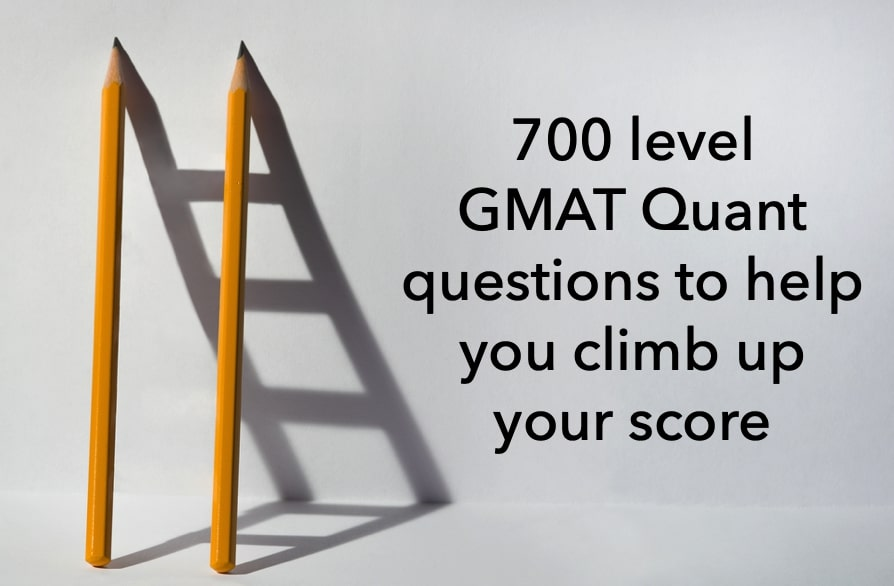Do you want to score a Q50/51 on GMAT Quant? To ace GMAT Math you should first master the 6 process skills and then practice a few GMAT Quant questions. Therefore, before proceeding with understanding these questions, we’d recommend you take a look at this article on Quant process skills first. If you have already gone through the first article then go ahead with the below questions.Here are 6 GMAT Quant questions to improve your score:

## GMAT Quant question 1

### Process Skill tested – “Infer”

All boxes in a certain warehouse were arranged in stacks of 12 boxes each, with no boxes left over. After 60 additional boxes arrived and no boxes were removed, all the boxes in the warehouse were arranged in stacks of 14 boxes each, with no boxes left over. How many boxes were in the warehouse before the 60 additional boxes arrived?

• There were fewer than 110 boxes in the warehouse before the 60 additional arrived.
• There were fewer than 120 boxes in the warehouse after the 60 additional arrived.

Now review the solution to this question. If you prefer a video solution then take a look at this video.

## Solution to Question 1

### Inference Process Skill at Play

Observe carefully what would have happened if you had not drawn the inference that x+5 is a multiple of 7.  You would have still arrived at the fact that statement 1 is not sufficient, but you would have also considered that statement 2 is not sufficient since x could be 1, 2, 3, or 4.  Even by combining the two statements, you would have concluded that the information is not sufficient, thereby selecting choice E as the correct answer.

Thus, drawing inference is the key to solving this question.

Now let’s look at how we arrived at the inference.

1. Information from the processing of the question statement.
• 6 (x + 5) = 7y
2. Required Conceptual Knowledge
• 6 is not a multiple of 7.
• Understanding of product of two numbers.
3. Inference
• Since 6 is not a multiple of 7, given the relationship, (x+5) has to be a multiple of 7.

We will discuss this skill in further detail in the subsequent articles that follow.

For now, review the definition of “Infer” once again.

“Given the information in the question and given your conceptual knowledge, what can you deduce so that you come closer to the solution of the question.”

Did you know a GMAT score of 730+ yields incremental \$500K in ROI? Start your GMAT Preparation by Signing up for our FREE Trial and get access to FREE online GMAT preparation resources. We are the most reviewed GMAT preparation company on GMATClub with more than 1870 reviews.

## GMAT Quant question 2

### Process Skill tested – “Translate”

If x is a positive integer, how many positive integers less than x are divisors of x?

• x2 is divisible by exactly 4 positive integers less than x2.
• 2x is divisible by exactly 3 positive integers less than 2x.

Now review the solution to this question. If you prefer a video solution then take a look at this video.

## Solution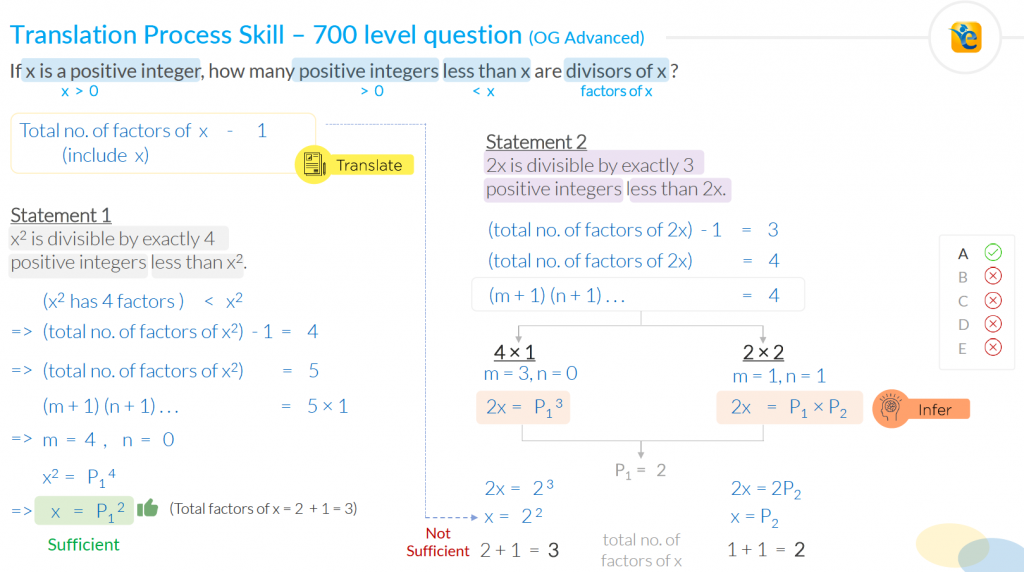### Translation Process Skill at Play

Observe the solution and notice how we read the question statement to determine what the question is asking us. For your kind perusal, we have presented the details of how we did the translation.

­­­­­­­­­­­­­­­­­­­­­

If x is a positive integer, how many positive integers less than x are divisors of x?

• If x is a positive integer,
• x > 0
• how many positive integers less than x
• need the number of integers
• these integers < x
• are divisors of x?
• These integers are factors of x
• We need the number of factors of x but these factors should be less than x.
• Total number of factors of x – 1
• since the total number of factors of x also includes the number x itself
• Notice that you are using your conceptual understanding of “the total number of factors”.

We want to draw your attention to the following points:

1. Notice how we strategically paused to translate. We split this short question statement into 3 parts.  This is very important so that you don’t miss out on any critical information.
2. Notice how we brought in our conceptual understanding of the total number of factors of a number.
3. Notice how this translation is helped us arrive at the mathematical form of what the question is asking us. In the absence of this step, we would have been completely lost in the question statement.  And the individual statements would not have helped at all.

We will discuss this skill in further detail in the subsequent articles that follow.  But for now, we want you to actively start translating information by reading the question statement by strategically pausing.

For now, review the definition of “Translate” once again.

Converting English into Mathematical form and vice versa, utilizing your conceptual knowledge.

## GMAT Math question 3

### Process Skill tested – “Simplify”

For each positive integer k, let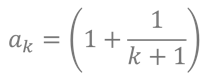Is the product a1a2…an  an integer?

• n + 1 is a multiple of 3.
• n is a multiple of 2.

Now review the solution to this question. If you prefer a video solution then take a look at this video.

## Solution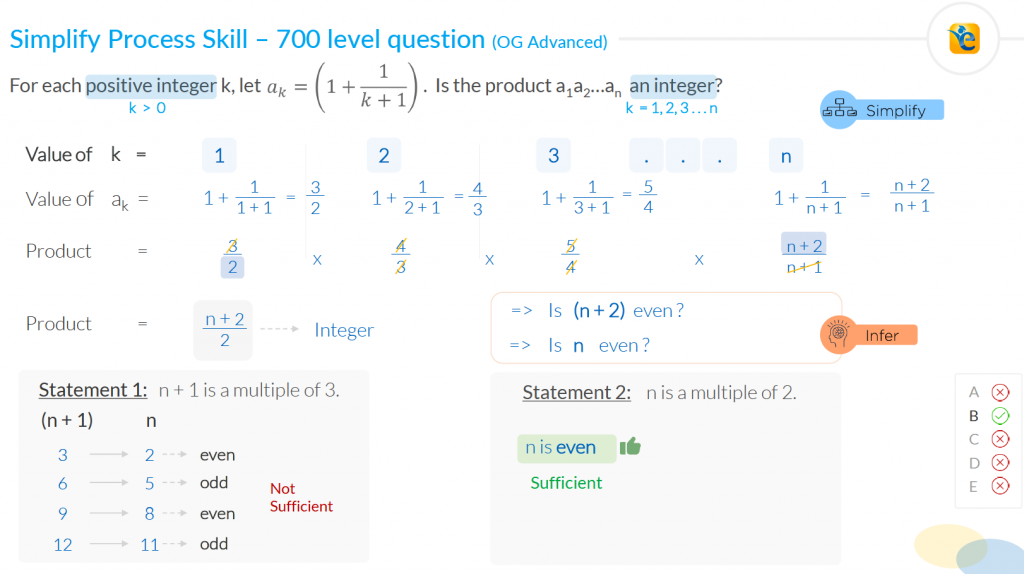### Simplify Process Skill at Play

Observe the solution and notice how while analyzing the question statement, we have taken such a complicated looking expression and very comfortably we simplified it into such a simple form – is n+2/2 an integer?

This is the process skill of “simplify” in action.

We want you to realize this fact – difficult GMAT Quant questions often contain complicated-looking expressions.  And we also want you to understand that the test maker will not throw anything at you that you cannot handle.  The way you do not have to be a grammarian to answer SC questions correctly, you do not need to be a mathematician to answer difficult quant questions correctly.

And this the reason for the importance of “simplify” process skill.  Whenever you see something scary and complicated, remind yourself that you have everything in your arsenal to tackle that complicated expression.  And you utilize the process skill of simplify.

At this point, I want to ask you a question – Look at the part where we simplify the question statement.  And tell me – is there any conceptual knowledge required to do this simplification?  The answer is no.  All that is needed is the comfort level in applying the process skill of “simplify”.

We will discuss this skill in further detail in the subsequent articles that follow.  But for now, we want you to not get scared of complex expressions but take them up head-on with the confidence that you will simplify them.

For now, review the definition of “Simplify” once again.

Bringing complicated-looking expressions into simple form.

## GMAT Math question 4

### Process Skill tested – “Visualize”

A border of uniform width is placed around a rectangular photograph that measures 8 inches by 10 inches.  If the area of the border is 144 square inches, what is the width of the border, in inches?

1. 3
2. 4
3. 6
4. 8
5. 9

Now review the solution to this question. If you prefer a video solution then take a look at this video.

## Solution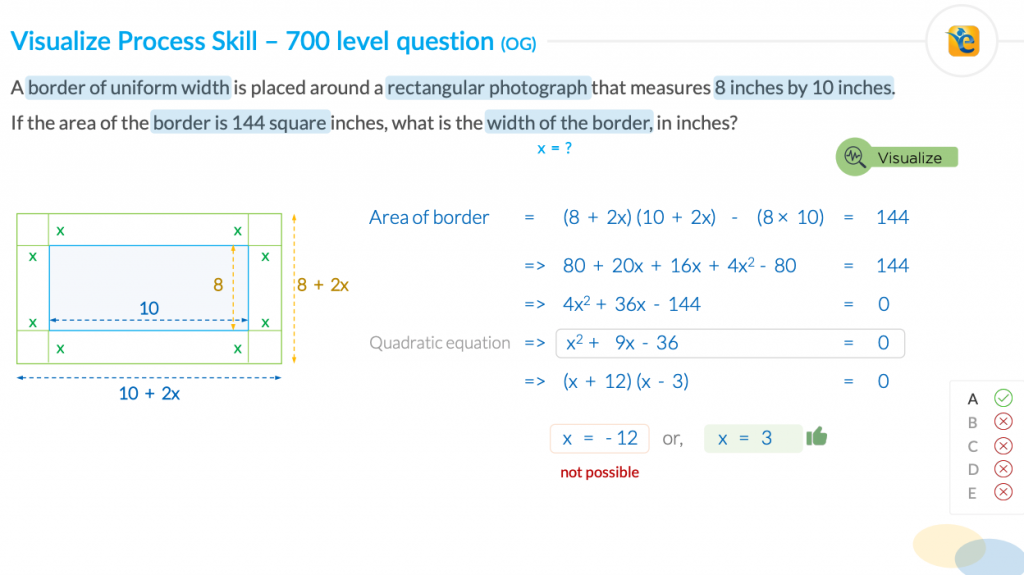### Visualize Process Skill at Play

It is very important to understand the question statement and convert it into a visual form so that we get more comfortable with the information provided.  Such visual aids enhance the clarity in our mind and help is get closer to the solution of the question.

So never give in to your laziness.  At the same time never feel overconfident that you can do it all in your mind.  Use your mind to do the processing and utilize these visual aids to make that processing more effective.

We will discuss this skill in further detail in the subsequent articles that follow.  But for now, we want you to consciously draw such diagrams and/or tables so that you get the level of clarity that you need to solve the question correctly.

For now, review the definition of “Visualize” once again.

Putting information in a visual form such as a figure, a table, a chart, a venn diagram, etc. to make the analysis easier.

## Question 5

### Process Skill tested – “Consider all cases”

Each entry in the multiplication table above is an integer that is either positive, negative, or zero. What is the value of a?

(1) h ≠ 0

(2) c = f

Now review the solution to this question.

## Solution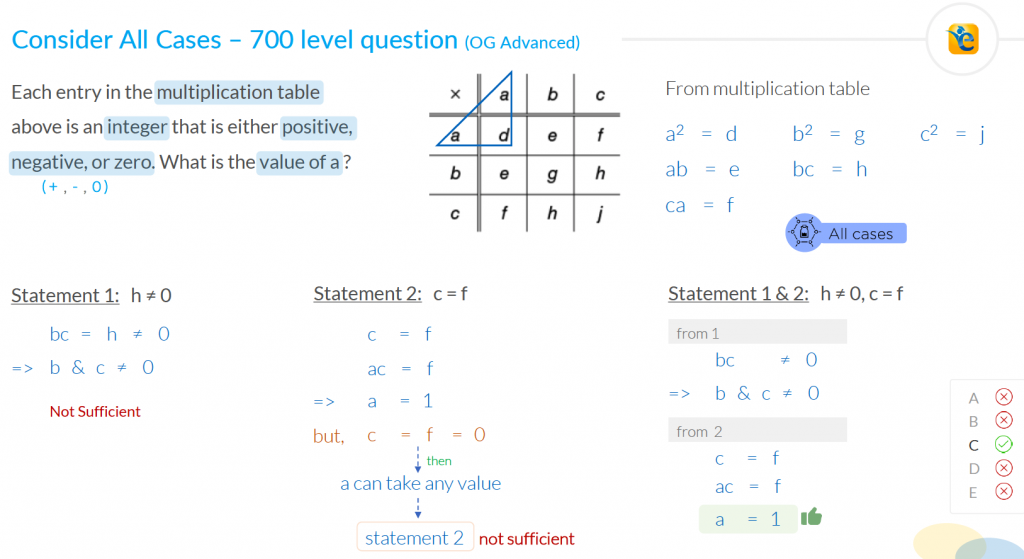### “Consider all Cases” Process Skill at Play

There are three things that I want to highlight, now that you have gone through the solution.

Critical process skill

I want to draw your attention to the analysis of statement 2.  You would agree with me that if you failed to consider the case in which c=f = 0, then you would arrive at incorrect answer choice.

Consider all cases is a very critical process skill that is often overlooked.  In fact, the test maker while framing difficult questions, relies on you not applying this process skill.

So, every test taker should work on building this process skill.

Conceptual understanding

Application of this skill has little to do with your conceptual understanding.  Its more about being aware of the confines that you are working with.

Feedback

Review the solution one more time and tell me – if you had made that mistake, what remedial action would you have taken?

Majority of students would have stated this mistake as a silly mistake and hence from a learning perspective, you would have lost an amazing opportunity to learn from this question.

On the contrary, if you started applying these process skills and you made this mistake, this e-GMAT solution would have pointed it out that you faltered here because you failed to apply the process skill of “consider all cases”.  Such precise feedback is what you need to improve as you solve the next set of questions.

This is the “magic” of applying process skills.  It not only helps improve your accuracy for medium and hard questions, but it also helps with improvement in ability as you incorporate such feedback.

We will discuss this skill in further detail in the subsequent articles that follow.  Next, we will talk about our last and definitely a very important process skill – Apply Constraints.

For now, review the definition of “Consider all Cases” once again.

While evaluating a relationship, we must consider all the applicable cases that could impact our evaluation of that relationship.

## Question 6

### Process Skill tested – “Apply constraints”

How many integers that satisfy the inequality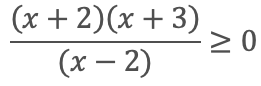are less than5?

1. 1
2. 2
3. 3
4. 4
5. 5

Now review the solution to this question.

## Solution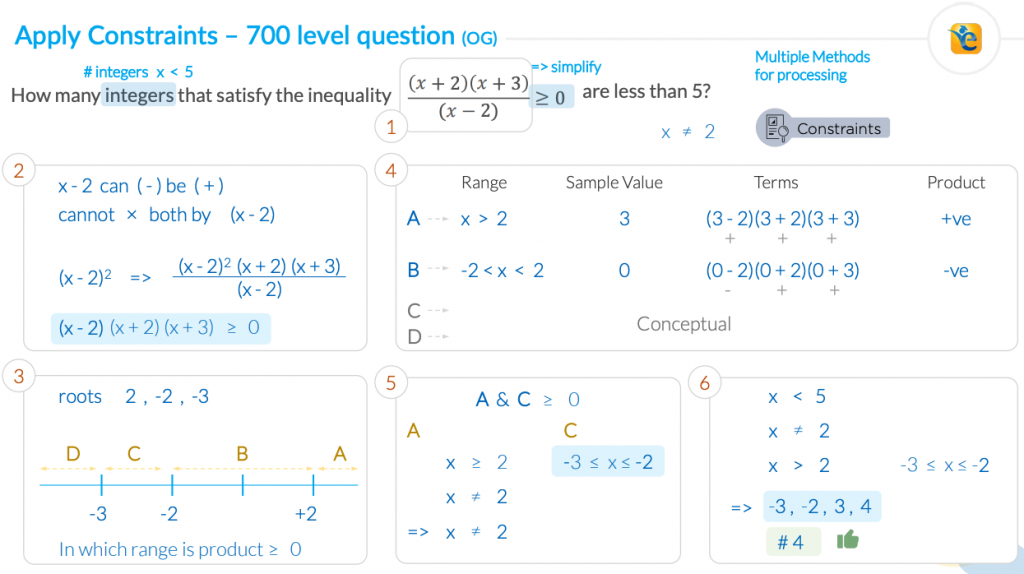### “Apply Constraints” Process Skill at Play

Notice carefully how we first simplified the inequality, and we brought it in a familiar form.  That was essential for the solution of the question.  At the same time, it was essential to note the constraints imposed on the system by the question statement.

The given inequality holds.  And this inequality has (x-2) in the denominator, so x cannot be 2 since the denominator cannot be zero.  You may wonder – how do I know when to do such analysis.  And the straightforward answer is that you do such analysis automatically when you start to apply the process skill of “apply constraints consciously”.

We will discuss this skill in further detail in the subsequent articles that follow.

For now, review the definition of “Apply Constraints” once again.

Consciously identify the constraints on variables in the question, based on the information in the question statement and based on your conceptual understanding.#### Payal Tandon

###### Browse Related Topics# Achieve 740+on the GMAT in 30 days!Sign up for our free trial and get400+ Practice questions with detailed solutions10+ hours of AI-driven video lessonsAdaptive mock test with ESR+ analysis